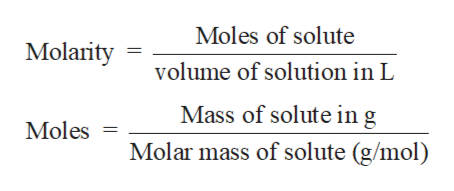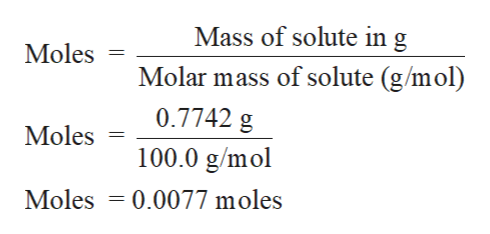# how would i determine the concentration of carbonate anion (CO32-) in g per L of a solution if there was 10.00 mL aliquot that yields 0.7742g of calcium carbonate

Question
9 views

how would i determine the concentration of carbonate anion (CO32-) in g per L of a solution if there was 10.00 mL aliquot that yields 0.7742g of calcium carbonate

check_circle

Step 1

Solution stoichiometry involves the calculation of concentration of solutions in the given conditions of volumes, moles etc.

Solution stoichiometry is mainly based on the calculation of moles and volumes. These two values are used to calculate the molarity of solution. The relation between moles, volume and molarity is as given below;help_outlineImage TranscriptioncloseMoles of solute Molarity volume of solution in L Mass of solute ing Molar mass of solute (g/mol) Moles fullscreen
Step 2

Mass of Calcium carbonate (CaCO3) =  0.7742 g

Volume of aliquot = 10.00 mL = 0.01 L

Molar mass of CaCO3 = 100.1 g/mol

Step 3

Calculate the moles of C...help_outlineImage TranscriptioncloseMass of solute in g Moles Molar mass of solute (g/mol) 0.7742 g Moles 100.0 g/mol Moles 0.0077 moles fullscreen

### Want to see the full answer?

See Solution

#### Want to see this answer and more?

Solutions are written by subject experts who are available 24/7. Questions are typically answered within 1 hour.*

See Solution
*Response times may vary by subject and question.
Tagged in
ScienceChemistry

### Solutions